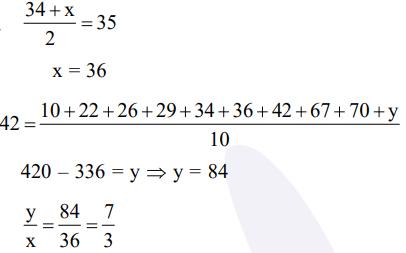# Solve this following`
Question:

The mean and the median of the following ten numbers in increasing order $10,22,26,29,34, x$

$42,67,70, y$ are 42 and 35 respectively, then $\frac{y}{x}$

is equal to :-

1. $7 / 3$

2. $9 / 4$

3. $7 / 2$

4. $8 / 3$

Correct Option: 1

Solution: